# 2019 Year 11 Physics Kinematics Practice Questions

The 2019 Year 11 Physics Kinematics Practice Questions are here for you. Test your Physics skills and identify your weaknesses before it's too late.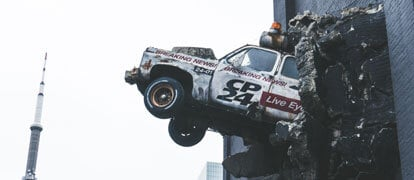## Year 11 Physics: Kinematics

Below are ten questions to test how well you know Kinematics for year 11 Physics.

You will find the answers to these questions here at the bottom of the page.

### Question 1 (4 Marks)

A rally driver is competing in a race. Initially heading north, the driver travels for $$10 \textrm{ minutes}$$ at $$100 \text{ kmh}^{-1}$$, before turning east and travelling $$5 \textrm{ km}$$ in $$4 \textrm{ minutes}$$. The driver then turns south and travels for $$3\textrm{ minutes}$$ at the same speed.

a) Calculate the velocity and displacement for each leg of the journey.

b) Calculate the final displacement of the driver, and the average velocity for the whole journey.

### Question 2 (3 Marks)

The velocity of a car as a function of time is shown on the graph below. The car starts at the origin.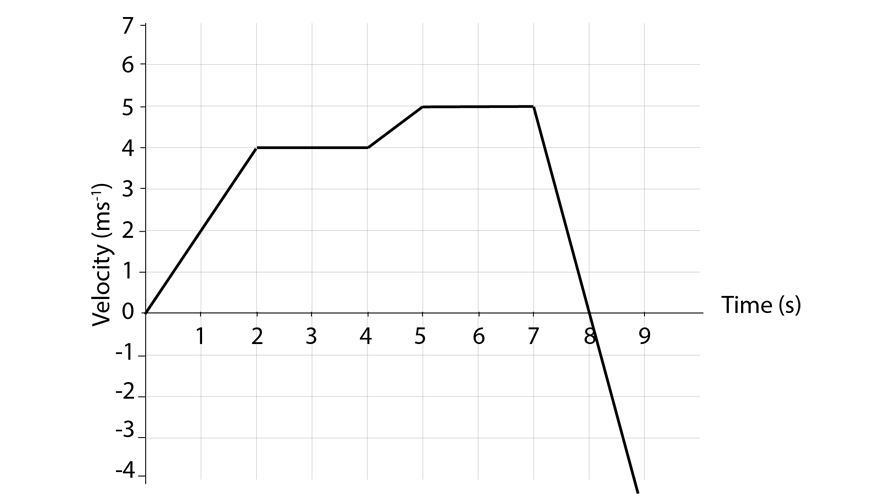a) Identify the time interval(s) where the car is moving backwards.

b) Calculate the instantaneous acceleration of the car at time $$t=1\textrm{ s}$$

c) Calculate the displacement of the car when it first stops.

### Question 3 (5 Marks)

You are observing the motion of two objects in a straight line. You record their positions every second, and gather the following data:

 Time (s) Object 1 position (m) Object 2 position (m) 0 0 5 1 0 5 2 2 4 3 4 3 4 5 3.5 5 4 3.5 6 3 2 7 3 0.5 8 3 0.5 9 3 1 10 2 1.5

a) Graph the displacement of each object as a function of time:b) Identify the object travelling with the higher speed at time $$t=2 \textrm{ s}$$.

c) Using the graph, estimate the time at which object 1 and object 2 are at the same position.

### Question 4 (5 Marks)

Calculate the final displacement and average velocity for the following objects:

a) An object is travelling to the right at $$15 \text { ms}^{-1}$$ for $$3 \textrm{ s}$$, then to the left at $$10 \textrm{ ms}^{-1}$$ for a further $$4 \textrm{ s}$$.

b) An object is released from rest in Earth’s gravity and falls under the influence of gravity only for $$5 \textrm{ s}$$.

c) An object is travelling at a speed of $$20 \textrm{ ms}^{-1}$$ forwards for $$2.4 \textrm{ s}$$, then accelerates backwards for $$4 \textrm{ s}$$ at $$5 \textrm{ ms}^{-2}$$

### Question 5 (5 Marks)

A police car is at rest, and observes a speeding sports car travelling past it at $$100 \text{ kmh}^{-1}$$. The police car gives chase and accelerates from rest at $$2.5 \text{ ms}^{-2}$$. For safety reasons, the police car can travel at a top speed of $$120 \text{ kmh}^{-1}$$.

a) Calculate the distance the police car travels in the time it takes to accelerate to $$120 \text{ kmh}^{-1}$$.

b) Calculate the duration of time it takes the police car to accelerate to $$120 \text{ kmh}^{-1}$$.

c) Calculate the distance the sports car travels in the time it takes the police car to accelerate to $$120 \text{ kmh}^{-1}$$.

d) The police car eventually catches up to the sports car. Calculate the time elapsed since the chase began.

### Question 6 (4 Marks)

Convert the following vectors into Cartesian form (perpendicular components). Assume the $$+x$$ direction is to the right, and the $$+y$$ direction is up.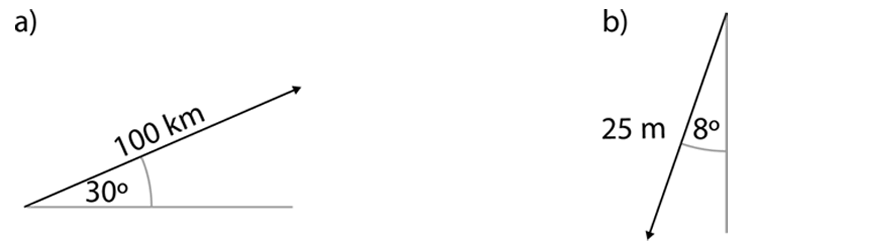Convert the following vectors into polar form (magnitude and direction). Assume north to be up the page.### Question 7 (3 Marks)

Perform the following vector operations: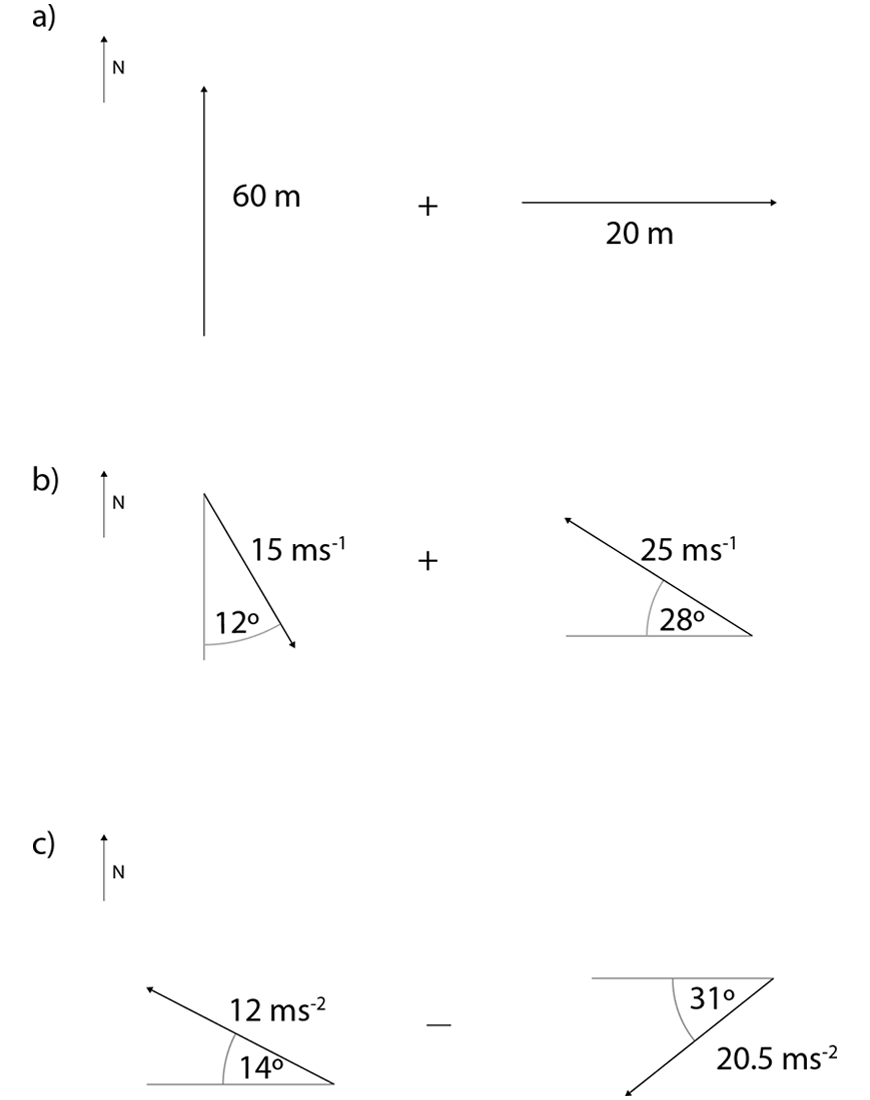### Question 8 (4 Marks)

A car is travelling at a speed of $$10 \text{ ms}^{-1}$$ due north when it enters a clockwise circular turn with a radius of $$5 \text{ m}$$. It then undergoes uniform circular motion around the turn.

a) Outline how the speed of the car changes as it traverses the turn.

b) Calculate the instantaneous (centripetal) acceleration of the car when it is in the turn.

c) The car exits the turn due east at the same speed as it entered. Calculate the average acceleration of the car.

### Question 9 (4 Marks)

An aeroplane is attempting to make a round-trip between cities that are $$300\text{ km}$$ apart, one directly north of the other. The cruising speed of the aeroplane is $$200 \text{ ms}^{-1}$$.

On the initial journey heading north, the aeroplane experiences a headwind of $$30\text{ ms}^{-1}$$.

a) What is the velocity of the aeroplane relative to the ground?

b) How long does it take the aeroplane to complete this part of the journey?

On the return journey heading south, the aeroplane experiences a crosswind of $$25\text{ ms}^{-1}$$ coming from the east.

c) Using a vector diagram, calculate the direction the aeroplane must head such that its resultant velocity is due south.

d) Calculate which leg of the journey is completed in the shortest amount of time.

### Question 10 (3 Marks)

Kevin rides his bike at $$7 \text{ ms}^{-1}$$ north. He sees a bird flying at $$10\text{ ms}^{-1}$$ south-west from his perspective.

a) Write an expression for the velocity of the bird relative to the ground in terms of Kevin’s velocity relative to the ground and the velocity of the bird relative to Kevin.

b) Using a vector diagram, calculate the velocity of the bird relative to the ground.

## Need more practice with Kinematics?

Be one step ahead of your peers with advanced completion of contents before it’s taught at school.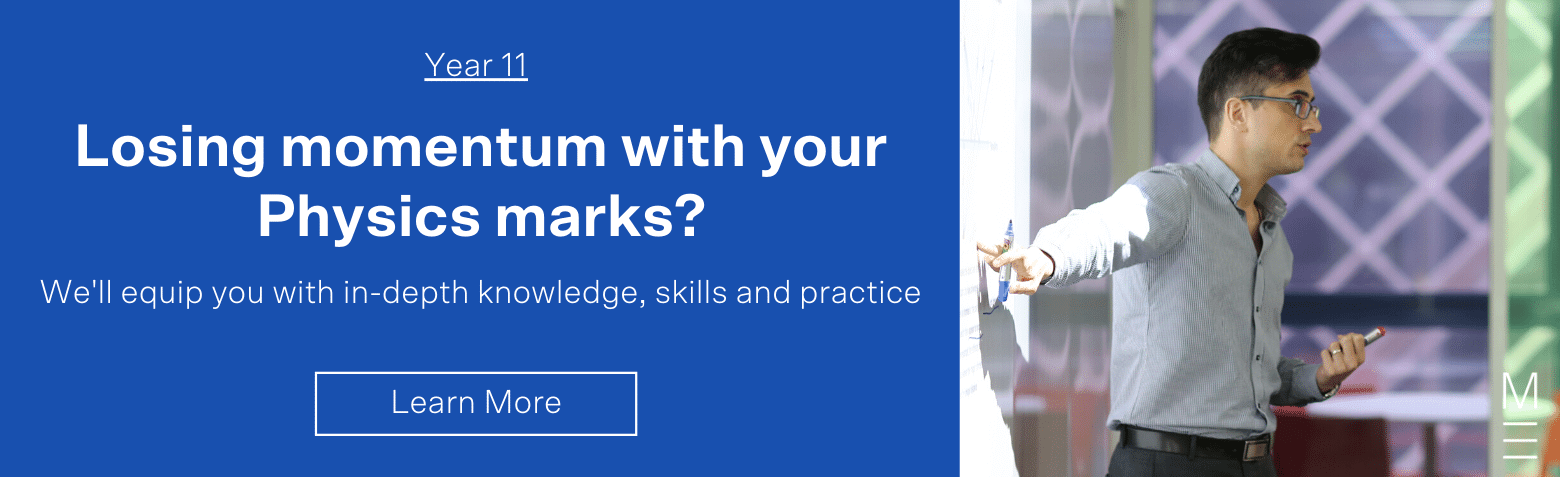## Solutions:

Question 1:

a) Leg 1: $$16.667 \text{km}, 100 \text{kmh}^{-1}$$
Leg 2: $$5 \text{km}, 75 \text{kmh}^{-1}$$
Leg 3: $$3.75 \text{km}, 75 \text{kmh}^{-1}$$

b) Displacement: $$13.85 \text{km, N}21.2^\circ E$$
Velocity: $$48\text{kmh}^{-1} \text{, N}21.2^\circ E$$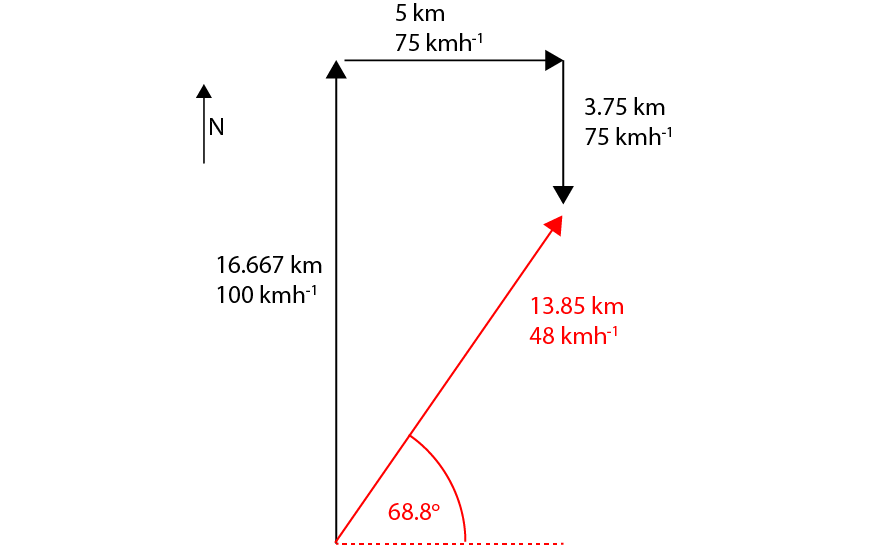Question 2:

a) $$t = 8 – 9 \text{ s}$$

b) $$2\text{ ms}^{-2}$$

c) $$29\text{ m}$$

Question 3:

a)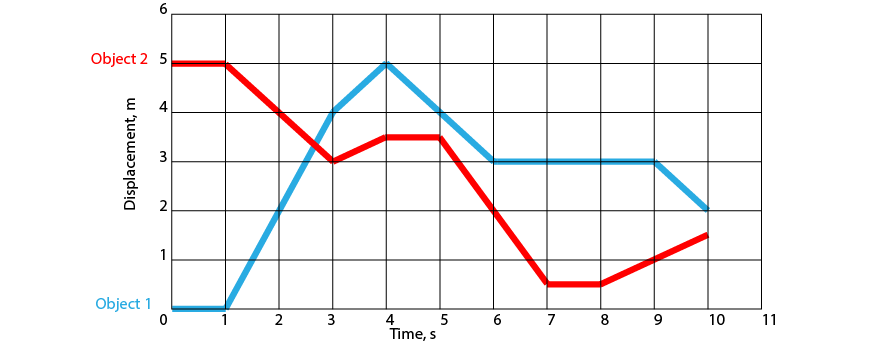b) Object 1

c) At approximately $$t=2.7\text{ s}$$

Question 4:

a) $$5 \text{ m}$$ and $$0.71 \text{ ms}^{-1}$$ to the right.

b) $$122.5 \text{ m}$$ and $$24.5 \text{ ms}^{-1}$$ down.

c) $$88 \text{ m}$$ and $$13.75 \text{ ms}^{-1}$$ forwards.

Question 5:

a) $$222 \text{ m}$$

b) $$13 \text{ s}$$

c) $$370 \text{ m}$$

d) $$40 \text{ s}$$

Question 6:

a) $$x = 86.6 \text{ km}, y = 50 \text{ km}$$

b) $$x = -3.48 \text{ m}, y = -24.76 \text{ m}$$

c) $$29.21 \text{ ms}^{-2}, \text{S38}^{\circ}\text{W or 218}^{\circ}\text{T}$$

d) $$17.32 \text{ ms}^{-2}, \text{S74.6}^{\circ}\text{E or 105.4}^{\circ}\text{T}$$

Question 7:

a)  $$63.25 \text{ m}, \text{N18.44}^{\circ}\text{E or 18.44}^{\circ}\text{T}$$

b)  $$19.18 \text{ ms}^{-1}, \text{S81.2}^{\circ}\text{W or 261.2}^{\circ}\text{T}$$

c)  $$14.71 \text{ ms}^{-2}, \text{N23.8}^{\circ}\text{E or 23.8}^{\circ}\text{T}$$

Question 8:

a) The speed of the car does not change.

b) $$20\text{ ms}^{-2}$$ towards the centre of the circle.

c) $$18\text{ ms}^{-2}, \text{ S45}^{\circ}\text{E}$$

Question 9:

a) $$170\text{ ms}^{-1}$$ North

b) $$29.4\text{ minutes}$$

c) The aeroplane must travel $$\text{S7.2}^{\circ}\text{E, or 172.8}^{\circ}\text{T}$$d) Effective velocity in second leg is $$198.36 \text{ ms}^{-1}$$, giving a total travel time of $$0.42\text{ hours} = 25.2\text{ minutes}$$. The first leg has a travel time of $$29.4\text{ minutes}$$, and therefore the return journey took the least amount of time.

Question 10:

a) $$v_{BG} = v_{BK}+v_{KG}$$

b) $$7.07 \text{ ms}^{-1}\text{S89.4}^{\circ}\text{W}$$